Question

How many grams of O2(g) are needed to completely burn 35.9 g of C3H8(g)? C3H8 + 5O2 ---> 3CO2 + 4H2O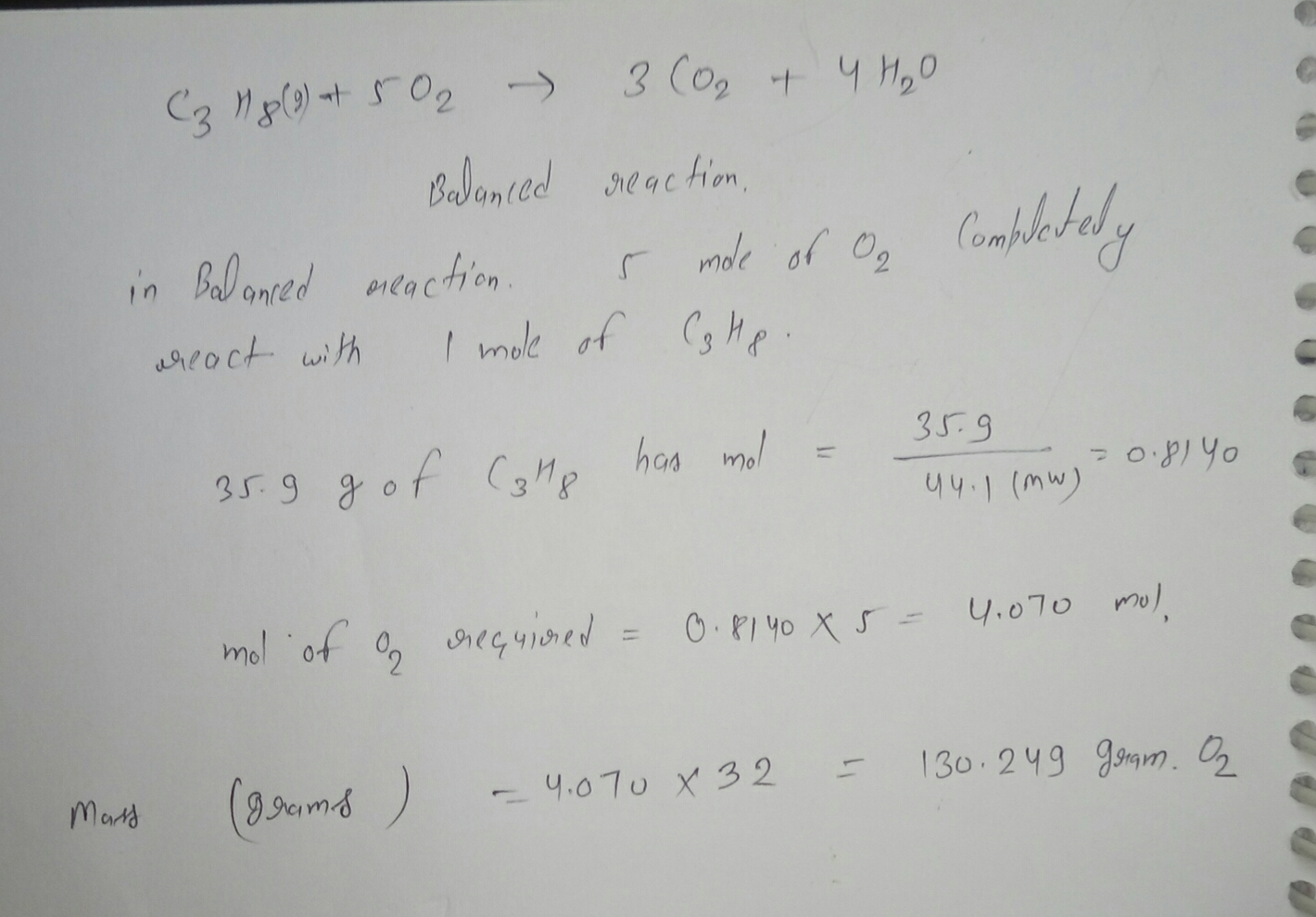#### Earn Coins

Coins can be redeemed for fabulous gifts.

Similar Homework Help Questions
• ### The combustion of propane may be described by the chemical equation, How many grams of O2(g)...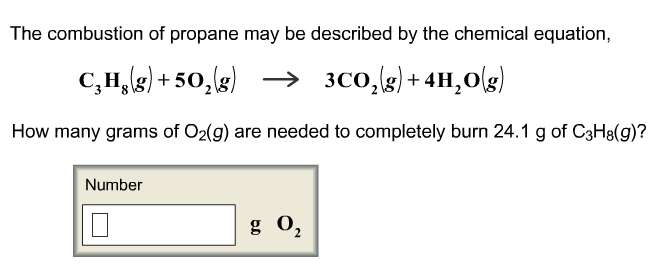The combustion of propane may be described by the chemical equation, How many grams of O2(g) are needed to completely burn 24.1 g of C3H8(g)? Number

• ### The combustion of propane may be described by the chemical equation, C3H8(g) + 5%g) -> 3C02(g)...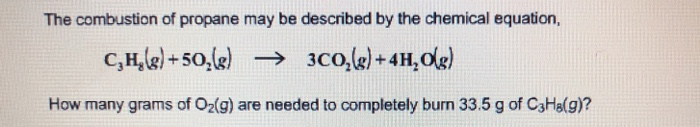The combustion of propane may be described by the chemical equation, C3H8(g) + 5%g) -> 3C02(g) +4H2 How many grams of O2(g) are needed to completely burn 33.5 g of C3H8(g)?

• ### If we have C3H8 + 5O2 ---> 3CO2 + 4H2O a) How many liters of oxygen...

If we have C3H8 + 5O2 ---> 3CO2 + 4H2O a) How many liters of oxygen gas are required to react with 7.2 L of C3H8 if both gasses are at STP? b) How many grams of CO2 will be produced from 35 L C3H8 at 15 C and 1.65 atm? c) How many grams of water vapor can be produced when 15 L C3H8 at 15 C and 1.65 atm are reacted with 15 L O2 at -20.0 C...

• ### The combustion of propane (C3H8) produces CO2 and H2O: C3H8 (g) + 5O2 (g) → 3CO2...

The combustion of propane (C3H8) produces CO2 and H2O: C3H8 (g) + 5O2 (g) → 3CO2 (g) + 4H2O (g) The reaction of 7.5 mol of O2 with 1.4 mol of C3H8 will produce ________ mol of CO2. Group of answer choices.

• ### CsH8 + 5O2- → 3CO2 + 4H3O How many grams of oxygen are required to burn...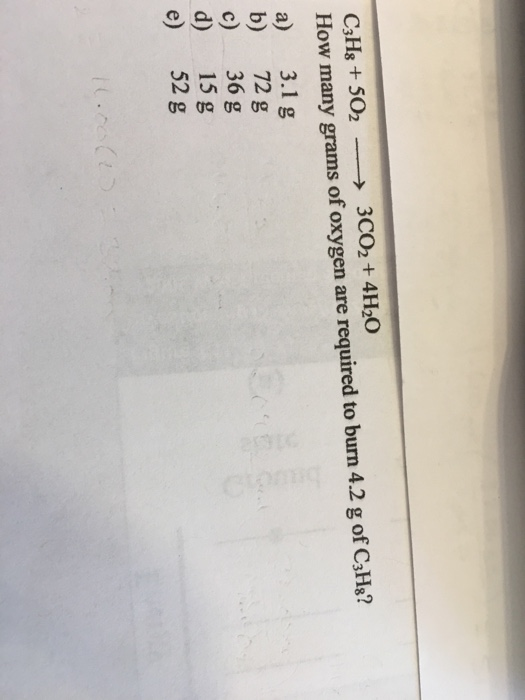CsH8 + 5O2- → 3CO2 + 4H3O How many grams of oxygen are required to burn 4.2 g of CaHa? a) 3.1 g b) 72 g c) 36 g d) 15 g e) 52 g

• ### Please help? Calculate deltaHr for the reaction: C3H8(g) + 5O2(g)----->3CO2(g) + 4H2O(l) Given: 3C(s) + 4H2(g)----->C3H8(g)...

Please help? Calculate deltaHr for the reaction: C3H8(g) + 5O2(g)----->3CO2(g) + 4H2O(l) Given: 3C(s) + 4H2(g)----->C3H8(g) deltaH -24.8 kcal    H2(g) + 1/2 O2(g)----->H2O(l) deltaH -68.3kcal C(s) + O2(g)-------> CO2(g) deltaH -94.0 kcal

• ### Consider the following reaction: C3H8+5O2→3CO2+4H2OC3H8+5O2→3CO2+4H2O What is the reducing agent? C3H8C3H8 CC OO O2

Consider the following reaction: C3H8+5O2→3CO2+4H2OC3H8+5O2→3CO2+4H2O What is the reducing agent? C3H8C3H8 CC OO O2

• ### Question (1 point) 1st attempt How many moles of O2 would be needed to cause the...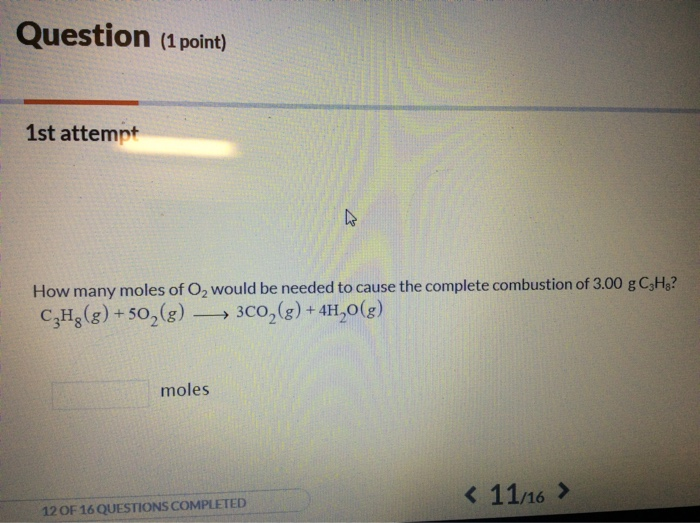Question (1 point) 1st attempt How many moles of O2 would be needed to cause the complete combustion of 3.00 g C,Ha? C3H8 (g) + 502 (g) → 3CO2 (g) + 4H2O(g) moles 16 12 OF 16 QUESTIONS COMPLETED

• ### 4 Na(s) + O2(g) → Na2O(s) a. How many grams of sodium are needed to completely...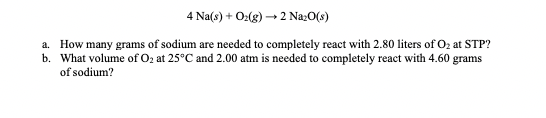4 Na(s) + O2(g) → Na2O(s) a. How many grams of sodium are needed to completely react with 2.80 liters of Oz at STP? b. What volume of O, at 25°C and 2.00 atm is needed to completely react with 4.60 grams of sodium?

• ### ( a) In the complete combustion of propane, how many moles of H2O(l) are produced per mole of CO2(g)? (b) A 1.638-g sample of propane is burned in excess oxygen. What are the theoretical yields (in grams) of CO2(g) and H2O(l) expected from the reaction

C3H8(g)+5O2(g)→ 3CO2(g)+4H2O(l)